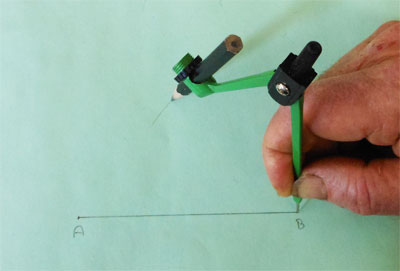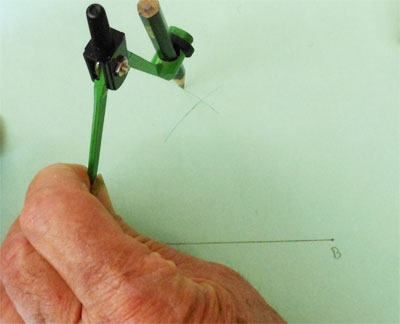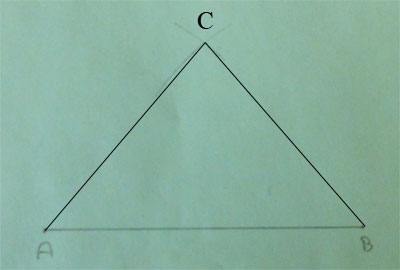SEARCH HOMEMath Central Quandaries & QueriesQuestion from Izzy, a student: what's the height of an isosceles triangle which has a base of 50 m, and both of the other sides are 25 m?Hi Izzy,

I changed the scale in order to fit a diagram on a piece of paper. I want to construct an isosceles triangle with a base of length 10 cm.

Draw a line segment of length 10 cm. Label the end points A and B. Set the distance between the point and pencil point of a compass to be 8 cm. Place the point at B and draw an arc above the midpoint of AB.Without changing the setting on the compass place the point at A and draw an arc to intersect the first arc.Label the point of intersection C and join A to C and then B to C.You now have an isosceles triangle with base of length 10 cm and the other two sides of length 8 cm.

Set the compass so that the distance between the point and the pencil point is 4 cm. Perform the same construction as above. What can you say about an isosceles triangle with base of length 10 cm and the other two sides of length 4 cm?

Set the compass so that the distance between the point and the pencil point is 5 cm. Perform the same construction as above. What can you say about an isosceles triangle with base of length 10 cm and the other two sides of length 5 cm?

PennyMath Central is supported by the University of Regina and The Pacific Institute for the Mathematical Sciences.